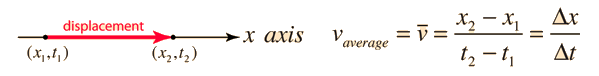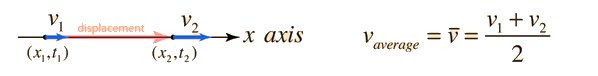# Velocity

The average speed of an object is defined as the distance traveled divided by the time elapsed. Velocity is a vector quantity, and average velocity can be defined as the displacement divided by the time. For the special case of straight line motion in the x direction, the average velocity takes the form:The units for velocity can be implied from the definition to be meters/second or in general any distance unit over any time unit.

You can approach an expression for the instantaneous velocity at any point on the path by taking the limit as the time interval gets smaller and smaller. Such a limiting process is called a derivative and the instantaneous velocity can be defined asAverage velocity, straight line motion Average velocity, general case
Index

Motion concepts

 HyperPhysics***** Mechanics R Nave
Go Back

# Average Velocity, Straight Line

The average speed of an object is defined as the distance traveled divided by the time elapsed. Velocity is a vector quantity, and average velocity can be defined as the displacement divided by the time. For the special case of straight line motion in the x direction, the average velocity takes the form:If the beginning and ending velocities for this motion are known, and the acceleration is constant, the average velocity can also be expressed asFor this special case, these expressions give the same result.

### Example for non-constant acceleration

Index

Motion concepts

 HyperPhysics***** Mechanics R Nave
Go Back

# Average Velocity, General

The average speed of an object is defined as the distance traveled divided by the time elapsed. Velocity is a vector quantity, and average velocity can be defined as the displacement divided by the time. For general cases involving non-constant acceleration, this definition must be applied directly because the straight line average velocity expressions do not work.If the positions of the initial and final points are known, then the distance relationship can be used to find the displacement.
Index

Motion concepts

 HyperPhysics***** Mechanics R Nave
Go Back